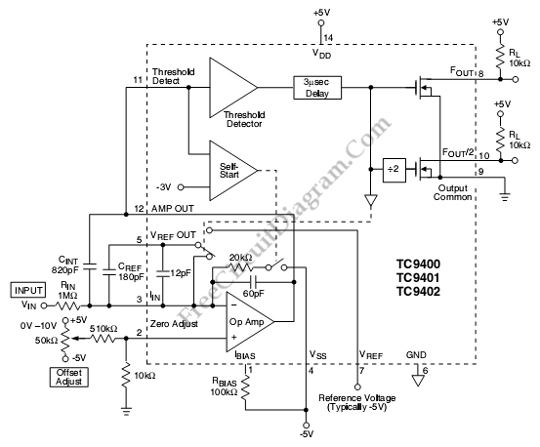## Pulse Delay Generator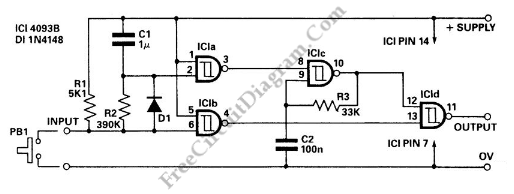This circuit is a Delayed Pulse Generator that is used to provide pulse rate and independent control of initial delay. The pulse generator of this circuit is IC1c which the operation is depended on  the normally low O/P of the IC1a. The PB1 is used to make the circuit input goes low. When PB1 is pressed, IC1b O/P goes high […]

## Accurate Low-Cost Square-Root Converter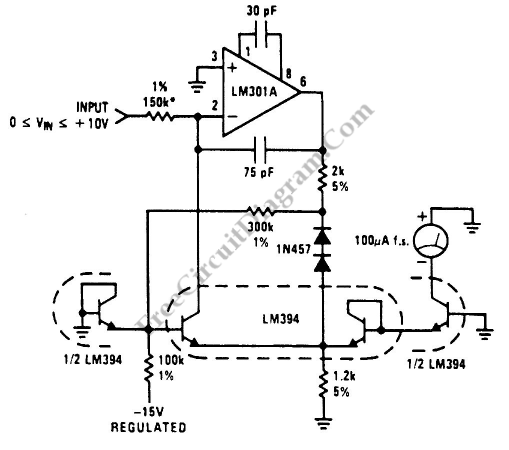This is a Low-Cost Accurate Square-Root Circuit. This circuit is used to provide a square-root function with a good accuracy. The benefit of This circuit is cheap. This circuit produce a current output which can be used to be converted to voltage by a summing junction current-to-voltage converter or to drive a meter directly. This circuit requires 15V supply. The […]

## Low Distortion Crystal Oscillator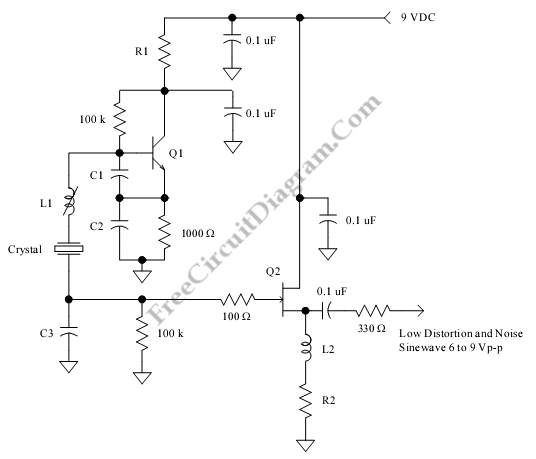This is a Low Distortion Crystal Oscillator circuit. This circuit generate a sinewave that has low phase noise and distortion. This circuit can be used to perform a crystal with less than 1mV dissipated in crystal. The crystal is used to filter the signal current. If the impedance loads is low, the JFET will drive the impedance. when the loads […]

## Voltage Controlled Oscillator with Varactor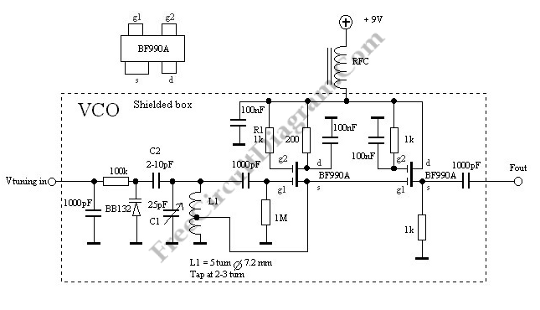This is a Voltage Controlled Oscillator  (VCO) circuit. This circuit is based on Hartley oscillator. The frequency depend on the value of C1 and L1. The oscillation-frequency will change as the change of the capacitance in the varactor BB132 by The Vtuning voltage. The maximum frequency that can be changed is determined by th value of C2. For large frequency […]

## Frequency Source with Digital Control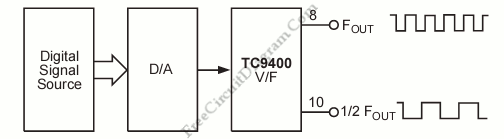This is a frequency source system that is controlled by a digital (microprocessor/microcontroller) system. This circuit produces  a square wave signal with adjustable frequencies. Here is the block diagram of digitally controlled frequency source system: This frequencies are controlled by microcontroller register, counter or thumb-wheel switches. This system can be used in many applications like numerically-controlled machine tools and computer-controlled […]

## TC9400 Frequency-to-Voltage Converter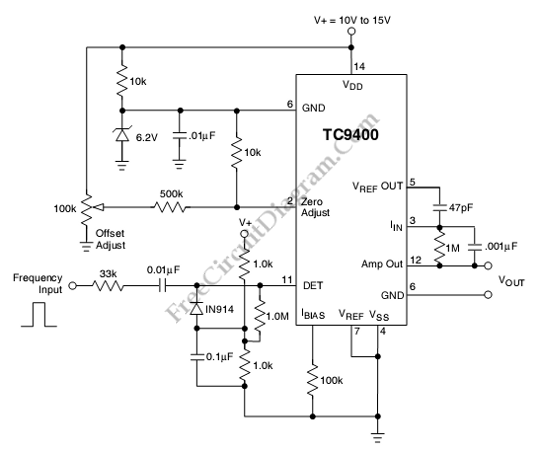This is a TC9400 Frequency-to-Voltage Converter circuit. Beside used as voltage to frequency converter, the TC9400 can also used as Frequency-to-Voltage Converter. The TC9400 will convert the input frequency waveform to output voltage linearly. This circuit has a threshold detector’s input that is used to detect each zero. The zero will cause a precise amount of charge(q=Cref Vref) to be […]